Astronomers have mapped out the sky in the shape of a spherical dome the Celestial Sphere, where earth is just a tiny spec at the center of the dome. The celestial sphere is divided into declination and right ascension just like earthâ€™s latitude and longitude lines.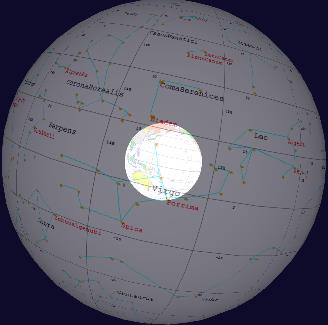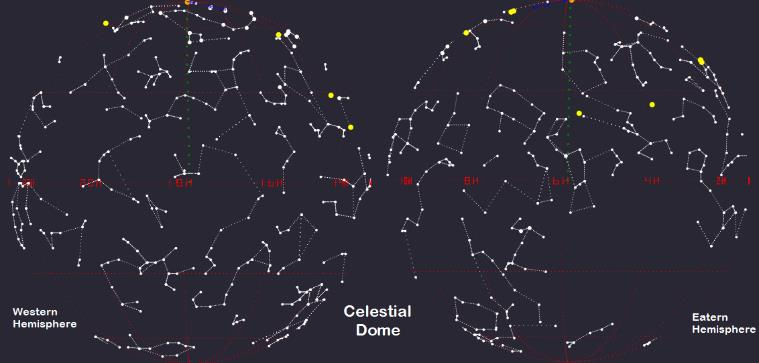Stars are identified by the coordinates of Declination and Right Ascension and are assumed to be fixed as their position donâ€™t change on day to day basis. Table 1 provides a list of major constellations and star coordinates.
For every Declination point in the sky there is a corresponding Latitude on earth but Longitude and Right Ascension coincide only for a moment each day and drift away as earth spins about its axis. This also means that while the earth equator and celestial equator are on the same plane, the prime meridian and 0o Right Ascension planes are the same only once a day.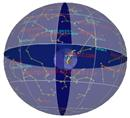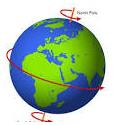## Where is 0o Right Ascension?

Since the earth is constantly spinning, astronomers had to choose a fixed reference point in time where the 0o Right Ascension line coincides with the 0o Longitudinal line.
The time they chose was the Vernal Equinox of March 20, 2000, at 7:30 AM Greenwich Mean Time (GMT).

Right Ascension Offset

Note

Notes

## Right Ascension Offset

A single rotation of earth about its axis is a little short of 24 hours. It is 23 hours, 56 minutes and 4 seconds or 86164 seconds (also called sidereal time).
You can easily calculate Right Ascension offset from any reference longitudinal line, simply compute the total number of turns earth has rotated since the 2000 Vernal Equinox, ignore the complete turns but add the remainder degrees to your current location longitude. This value added to the longitude is the reference point Right Ascension.
09/29/2017 2:30 AM - 03/20/2000 7:30 AM = 553114800 seconds.
Get time difference in seconds from the following URL:
(http://www.SahraID.com/MathAstrononmy)
Earth revolution: 553114800 / 86164 = 6419 turns plus 117 degrees.
117 + (-91) = 26. The reference longitude for Baton Rouge, LA is 26o.
If you were to draw an arrow from the center of the earth to a reference latitude and longitude then extending it into the sky is the respective Declination and Right Ascension angle.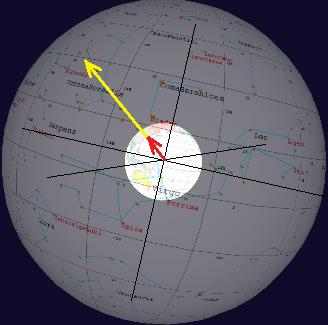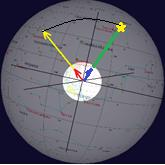Stars are arc length away from the point of observation as length and direction of arc can be computed geometrically.

Great Circle Arc

Note

Notes

## Great Circle Arc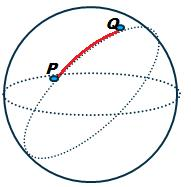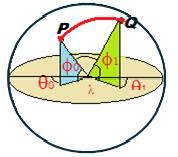## North Pole as reference direction

A reference point R can be added (equal in length of the arc) in the direction of north by simply adding arc length to the latitude of the point of observation P and the angle ï¡ is obtained using the dot product rule as explained in the Workshop section.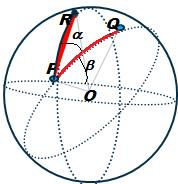## 3D Compass as a pointing device

3DCompass is a tool to point to the direction of a star in the 3-Dimensional space. The Compass is made of two semi-circle protractors, one for pointing to the Declination and the other for the Right Ascension. The Declination half has a magnetic needle pointing to North and the Right Ascension has a sliding Arrow pointer providing direction in the Sky where star is located.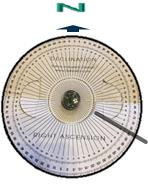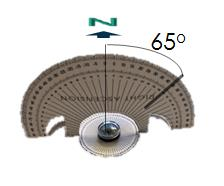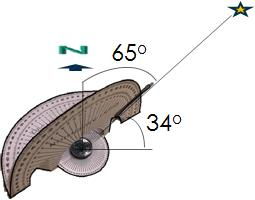As mentioned earlier only the angle of Right Ascension and the Declination relative to your reference location is sufficient to locate a star.
Just like latitude and longitude are sufficient to point to any place on earth, similarly, Right Ascension and Declination are sufficient to point to any place in the Celestial Hemisphere. The two coordinate systems can be made to coincide with each other by taking in to consideration the degrees of shift in the Right Ascension by earth rotation.

How to use 3D Compass

Note

Notes

## How to use 3D Compass

Table 1. Star Coordinates and 3D Compass Arc angles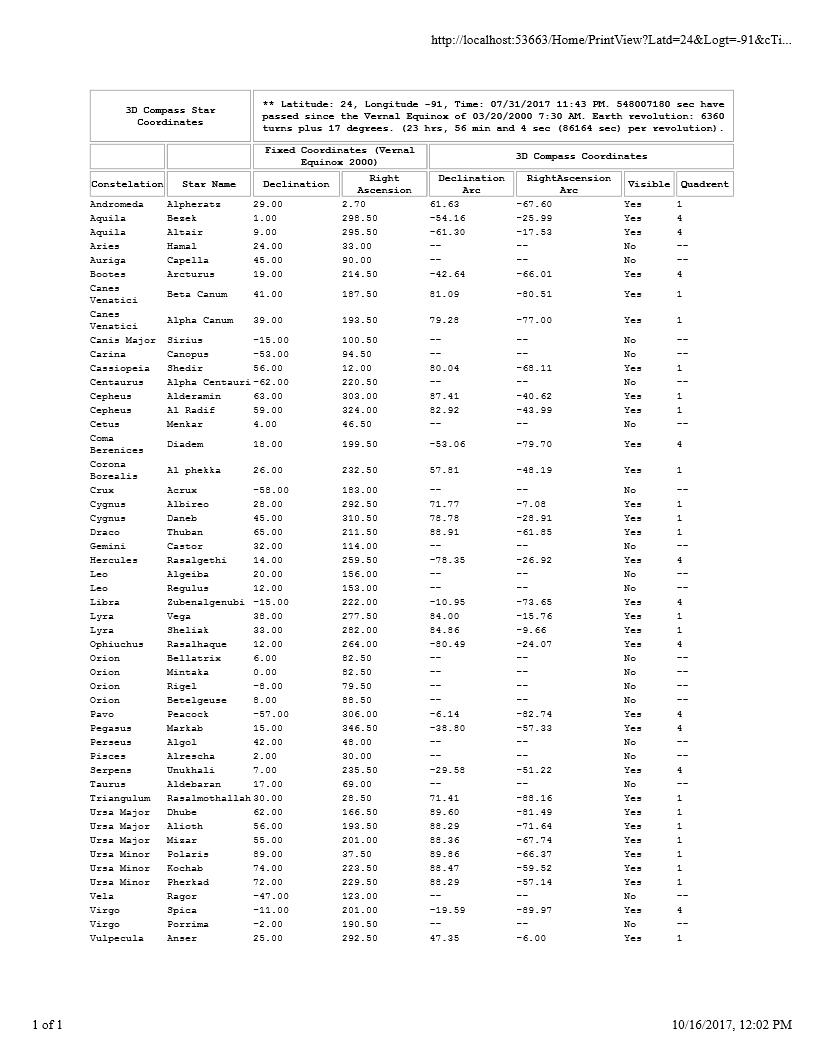Workshop

Note

Notes

## Workshop

Exercise 1
Calculate number of revolutions earth has made since Vernal Equinox of Mar 20, 2000 7:30 AM (GMT) till September, 29, 2:30 AM. (earth takes 23 hours, 56 minutes and 4 second to revolve around its own axis.)
9/20/2017 2:30 AM â€“ 3/20/2000 7:30 = 553622400 seconds.
Earth revolution: 553114800 / 86164 = 6419 20 turns plus 117 degrees.
Exercise 2
Calculate the Arc length ï¬ of star Betelgeuse from earth latitude 30o, longitude -91o at the time October, 4, 11:30 PM. (The celestial coordinates of star Betelgeuse are 8o Declination, 88.5o Right Ascension.) The Arc indicates the Right Ascension angle for 3D Compass.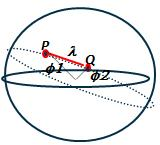Earth has shifted 117o from Celestial coordinates (see Exercise 1).
Reference longitude = -91 + 117 = 26o, Reference latitude = 30o. Point P (30,26).
Star Declination = 8o, Star Right Ascension 88.5o. Point Q (8, 88.5).
Î”Ï† = cos-1(cos(Ï†1) x cos(Ï†2) x cos(Î») + sin (Ï†1) x sin(Ï†2))
Î”Ï† = cos-1(cos(30) x cos(8) x cos(88.5-26)) + sin (30) x sin(8))
Î”Ï† = 62.5o

Exercise 3
Draw a point facing north equal distance of arc length from reference location (30,26) to star coordinate (8,88,5).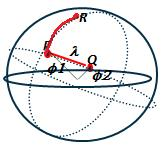Simply add the arc length 62.5o (from exercise 2) to the reference latitude.
Point R = ((30 + 62.5), 26) = 92.5o,26o
Exercise 4
Convert polar coordinates of point P, R and Q into rectangular coordinates
Point P (26,30),
Px = cos(30)x(cos(26) = 0.78, Py=cos(30)xSin(30) = 0.38, Pz = Sin(30) = 0.5.
Point Q (8,88.5),
Qx = cos(8)x(cos(88.5) = 0.026, Qy=cos(8)xSin(88.5) = 0.99, Qz = Sin(8) = 0.14.
Point R (92.5,30),
Rx = cos(8)x(cos(88.5) = -0.04, Ry=cos(8)xSin(88.5) = -0.017, Rz = Sin(8) = 0.99

Exercise 5
Convert to Vector PR (point P and point R) and convert to Vector PQ (Point P and point Q)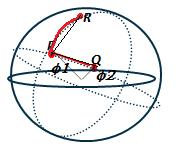Vector PR
X = Rx â€“ Px Y= Ry- Py, Z = Rz - Pz
X = -0.04 â€“ 0.78 = -0.81, Y = -0.017 â€“ 0.38 = -0.4 Z = 0.5
Vector PQ
X = Qx â€“ Px Y= Qy- Py, Z = Qz - Pz
X = 0.026 â€“ 0.78 = -0.75, Y = 0.99 â€“ 0.38 = 0.61 Z = -0.36

Exercise 4
Compute Vector magnitude PR and PQ.
Magnitude of Vector PR = x2+y2+z2=-0.812+-0.42+0.52=1.03
Magnitude of Vector PQ = x2+y2+z2=-0.752+0.612+-0.362=1.03
Exercise 5
Compute dot product of the Vector PR and PQ.

DotProduct = PRâ—PQ = PRx*PQx+PRy*PQy+PRz*PQz =
PRâ—PQ = -0.81*-0.75+0.4*0.61+0.5* -0.36=0.19

Exercise 5
Compute angle ï¡ between the Vector PR and PQ.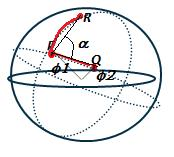Î±=cos-1dot productmagnitude A*Magnitude B
Î±=cos-10.191.03*1.03=80 degree
Exercise 5
Compute angle ï¢ between the vector PQ and the plane normal to the vector PR. (This is the declination angle for 3D Compass).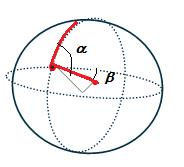ï¢ï€ = 90 - ï¡ï€ ï€½ï€ ï€¹ï€°ï€ ï€­ï€ ï€¸ï€°ï€ ï€½ï€ ï€±ï€°ï€ ï¤ï¥ï§ï²ï¥ï¥ï€